### RoHS Compliant Cable & Wire Technical Information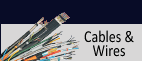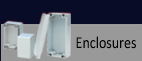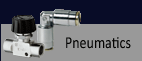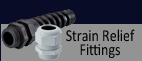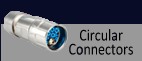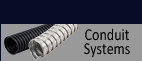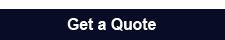*E-mail *Name *Company *Phone *State *Zip Code *Country
 Specify: P/N & Qty.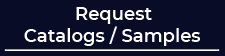303-680-5159 1-800-677-8942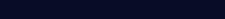## Formulas of Electric Engineering and Electronic

Cross-section for single wires round
 q = D² x π or   D² x 0.7854 4

Cross-section for bunched wires
 q = d² x π x n   or   d² x 0.7854 x n 4

Diameter for single wires
 D = √ q x 4 or   √q x 1.2732 π

Diameter for bunched wires
 D = √ 1.34 x n x d

q = Cross-section in mm²
D = Conductor diameter in mm
d = Single wire diameter in mm
n = Number of wires
Conductor resistance
 R = l or ρ x l κ x q q

 RCircuit = 2 x l or 2 x l x p κ x q q

R = Electrical direct current resistance Ohm
RCircuit = Resistance of complete circuit
q = Cross-section in mm²
κ (Kappa) = Conductivity
ρ (Rho) = Specific resistance
 (p = 1 ) κ
l = Conductor length in m
Materials Conductivity
 m Ω x mm²
Spec. Resistance
 Ω x mm² m
Copper 58.00 0.01724
Aluminum 33.00 0.0303
Silver 62.00 0.1613
Iron 7.70 0.1299
Constantan 2.00 0.50

Serial Connection
Resistance: R = R1 + R2 + R3 + ... + Rn

Capacitance:
 C = 1 + 1 + 1 + ... + 1 C1 C2 C3 Cn

Inductance: L = L1 + L2 + L3 + ... + Ln

Parallel connection
Resistance:
 R = 1 + 1 + 1 + ... + 1 R1 R2 R3 Rn

Capacitance: C = C1 + C2 + C3 + ... + Cn

Inductance:
 L = 1 + 1 + 1 + ... + 1 L1 L2 L3 Ln

Equivalent resistance of 2 parallel connections

 R = R1 x R2 R1 + R2

Mutual capacity
Coaxial cable:
 C = ξr x 10³ (nF/km) 18 x ln Da d

Parallel conductor:
 C = ξr x 10³ (nF/km) 36 x ln Da d

Shielded twisted pair:
 CB = ξr x 10³ (nF/km) 36 ln 2a x (Da²-a²) d x (Da²-a²)

 Da = Outer diameter over insulation Ds = Diameter over shield d = Diameter of conductor a = Distance - mid to mid of both conductors ξ = dielectric constant
Ohm's Law
The current intensity (I) is proportional to Voltage (U) and universaly proportional to Resistance (R)

 I = U I = U U = I x R R R
 I = Current intensity (in Ampere - A) R = Electrical resistance (in Ohm - Ω) U = Electrical Voltage (in V)
Conductance

 G = 1 S = 1 1μS = 1 R 1Ω 1MΩ
S (Siemens) = Reziprocal value of a resistance is used as conductance
S Siemens = 1/Ohm
G = Electrical conductance
Capacitance
Single conductor against ground

 CB = ξr x 10³ (nF/km or pF/m) 18 x ln Di d

Unshielded symmetrical twisted pair

 CB = ξr x 10³ (nF/km or pF/m) 36 x ln 2a d

Coaxial pair

 CB = ξr x 10³ (nF/km or pF/m) 18 x ln Di d

Shielded symmetrical twisted pair

 CB = ξr x 10³ (nF/km or pF/m) 36 ln 2a x (Da²-a²) d x (Da²-a²)
 Di = Outer diameter over single conductor Da = Outer diameter of multi conductors (mm) d = Diameter of conductor a = Distance - mid to mid of both conductors
Inductance of parallel conductors
at low frequencies

 L = 0.4 (In Da + 0.25) mH/km r

at high frequencies
 L = 0.4 (In Da + 0) mH/km r

Inductance of coaxial cable
at high frequencies

 L = 0.2 (In Da + 0) mH/km r
 Da = Distance - mid to mid of both conductors r = Radius of conductor ξr = dielectric constant
Impedance (Z)
for coaxial cable

 Z = 60 x In D (Ω) √ξr d

for communication cable
at low frequencies

 Z = √ R (Ω) x tan φ = 1, φ = 45° ωC

at high frequencies
 Z = √ L (Ω) C
 D = Diameter over insulation d = Conductor diameter R = Resistance (Ω/km) L = Inductance (mH/km) C = Capacitance (nF/km) ω = 2 π f
Wave Length
 λ = V f

Units of attenuation - Neper (Np), Decibel (dB) and Bel (B)
1 Np = 8.686 dB
1 dB - 0.1151 Np = 1/10 Bel
1 Bel = 10 dB = 1.1513 Np
 λ = Wave length V = Propagation velocity f = Frequency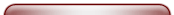Items For Quote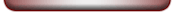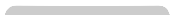Technical Information Copper & Alu Price Calculation Reference to DIN VDE Standards Harmonized Identification Code Designations Conductor Diameters and Resistance Strand Make-up acc. to DIN VDE 0295, IEC 60228 & HD 383 Nominal & Operating Voltage Current Carrying Capacity for Power Cables & Wires Installation Methods & Operating Conditions Laying Conditions for Power Cables Current Ratings for Installation Color Codes Bending Radius & Chemical Resistance Heat Resistance & Caloric Load Values Installation Instructions for UL and CSA Cables AWG Wires & AWG Stranded Conductors US and British Units - Conversions Current Ratings for UL-CSA Cables List of UL-Styles Index British Standard International Abbreviations Characteristics of Insulating and Jacket Materials Definitions: Classes of Stress in Flexible Cables and Insulated Wires Safety Requirements Glossary of Terms: Cables & Wires International Certification Marks & Testing Institute Formulas of Electronic & Power Engineering Cable-Drums - Capacity & Cable Lengths Notes on CE-Marking European Directives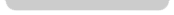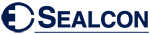is the Exclusive Importer of HUMMEL products. We offer over 6,000 different Types and Sizes of RoHS Compliant Liquid Tight Strain Relief Fittings, Cord Grips, Cable Glands, Circular Connectors, Conduit System, Industrial Enclosures and Other Related Cable Management Products which are rated the best in the industry.
7374 S. Eagle Street
Centennial, CO 80112-4221 USA
Toll Free: 800-677-8942 / 303-680-5159 FAX: 303-680-5344Top of Page